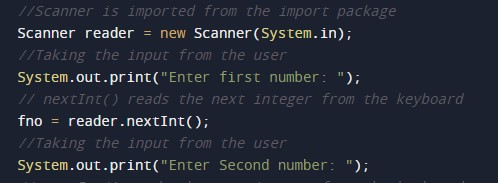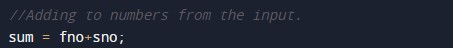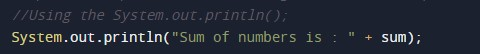# Java Program to Add Two Integer Number

In this tutorial you will learn about the Java Program to Add Two Integer Number and its application with practical example.

## Java Program to Add Two Integer Number

In this tutorial, we will learn to create a Java program that will Add Two Integer Numbers in Java programming.

## Prerequisites

Before starting with this tutorial, we assume that you are the best aware of the following Java programming topics:

• Operators in Java Programming.
• Basic Input and Output function in Java Programming.
• Basic Java Programming.

## Program to Add Two Numbers:-

In this tutorial, we will create a program that will add the two numbers using an addition operator. We will first take two numbers in input, and then we will add them and store the value in the third variable. At last, we will print the addition to the user.

With the help of this program, we can take input and Add Two Numbers.

## Output:-In the above program, we have first initialized the required variable.• fno = it will hold the input number value from the user.
• sno = it will hold the input number value from the user.
• sum = it will hold the sum value of the numbers.

Taking input numbers from the user.Calculating the sum of two numbers.Printing the output sum of two numbers.In this tutorial we have learn about the Java Program to Add Two Integer Number and its application with practical example. I hope you will like this tutorial.